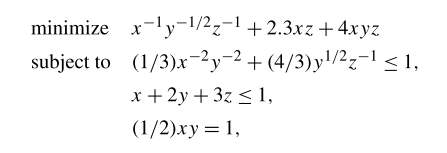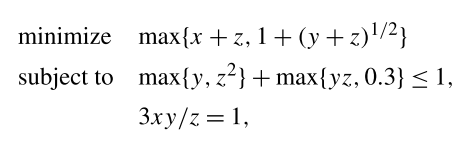# How to write equality constraints in GP？

Here is a GP in standard form：This is a simple problem, but I found some troubles. How can I express the equality constraint to make cvx work?
e.g. (1/2)xy = 1.
My code is follows:

``````cvx_begin gp
variable x
variable y
variable z
minimize x^(-1) * y^(-0.5) * z^(-1) + 2.3*x*z + 4*x*y*z
subject to
(1/3) * x^(-2) * y^(-2) + (4/3) * y^(0.5) * z^(-1) - 1 <= 0;
x + 2 * y + 3 * z - 1 <= 0;
(0.5) * x * y - 1 == 0;
cvx_end
``````

It’s wrong, can you tell me how to express equality constraints?

The error information is：

Disciplined convex programming error:
Invalid constraint: {convex} == {constant}

b = newcnstr( evalin( ‘caller’, ‘cvx_problem’, ‘[]’ ), x, y, ‘==’ );

(0.5) * x * y - 1 == 0;

In order to comply with GP mode rules:

Three types of constraints may be specified in geometric programs:
• An equality constraint, constructed using ==, where both sides are monomials.

change it to
`(0.5) * x * y == 1;`
That way, both sides of the equality are monomials, and comply with the above rule.

That being said, your problem appears to be infeasible, unless one or more of the values, 1, just before the `<=` are increased.

Thank you for your patience analysis. This example comes from Boyd’s “A tutorial on geometric programming”. I didn’t consider whether this problem can be solved. Thank you!

Its; interesting that the paper used an infeasible problem as its first example of a geometric program.- maybe the authors just wrote down something and didn’t bother checking feasibility. Nevertheless, it is a geometric program.

If you had stuck to entering the problem as the paper showed it, without trying to make the right-hand side equal to zero, CVX would have accepted it.

Thanks! Your answer is very detailed. Here I have another question, can’t CVX handle generalized posynomials inequality constraints? Here is a generalized geometric program (GGP) given by Stephen Boyd:Of course, it can be easily solved by converting GGP to GP, but in Boyd’s paper, he says,

In my opinions, I think it means that the Solver can convert GGP to GP automatically, both objective and constraints. However, Matlab gave me the error information:

clc
clear
cvx_begin gp
variable x
variable y
variable z
minimize max(x + z, 1 + (y + z)^0.5)
subject to
max(y, z^2) + max(y * z, 0.3) - 1 <= 0;
3 * x * y * z^-1 == 1;
cvx_end

Constraints may not involve internal, read-only variables.

b = newcnstr( evalin( ‘caller’, ‘cvx_problem’, ‘[]’ ), x, y, ‘<=’ );

max(y, z^2) + max(y * z, 0.3) - 1 <= 0;

The inequality constraint can’t be passed.
Later I tried another inequality constraints, such as:

Matlab shows：

``````  x + y + z - min(x^0.5 * y^0.5, (1 + x * y)^(-0.3) ) <= 0 ;
``````

Disciplined convex programming error:
Illegal operation: {log-convex} - {log-concave}

z = plus( x, y, true, cheat );

x + y + z - min(x^0.5 * y^0.5, (1 + x * y)^(-0.3) ) <= 0 ;

The inequality constraints still can’t pass. So, must I have to write constraints in the form of GP? Thanks.

You need to follow CVX’s rules. If you don’t like them, I suppose you are free to write your own GGP parser which is smarter and more flexible the CVX. Perhaps you can write a pre-parser for CVX which generates valid CVX code.

You can follow the rules in the CVX User’s Guide which allows modeling of GGPs having generalized posynomials, but these generalized posynomials don’t allow use of max (or min) in their formulation. The Boyd paper allows use of max in forming generalized posynomials, whereas CVX’s definition/rules don’t. I will have to defer to the CVX developer, mcg, for any further illumination on that.

But as you can see, you can follow the Boyd paper reformulation guidelines to create inputs acceptable to CVX. They can be GGPs, but apparently not quite as general as what Boyd considers to be GGPs.

Thanks, I think I can convert GGP to GP by myself. Thank you.